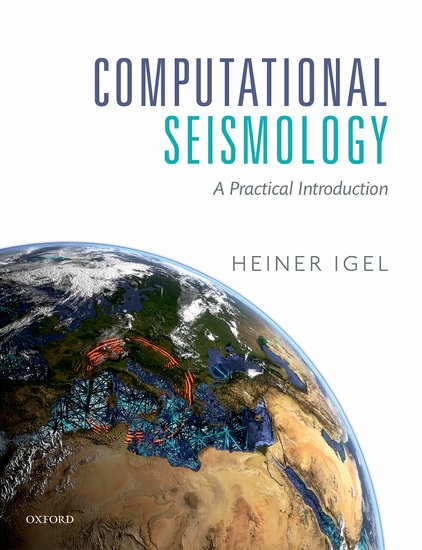Computational Seismology
Finite Volume Method - 1D Advection EquationThis notebook is part of the supplementary material to Computational Seismology: A Practical Introduction, Oxford University Press, 2016.

## Basic Equations¶

We want to solve the linear advection equation as a scalar hyperbolic equation:

\begin{equation} \partial_t q + a \partial_x q = 0 \end{equation}

This equation arises from conservation principles considering the case of advective fluxes. Let us partitioned the space in $n$ cells centered at $x = x_i$, the average of the quantity $q$ inside the cell is

\begin{equation} Q_{i}^{n} = \int_{\mathscr{c}} q(x,t) dx \end{equation}

Any change inside each cell implies a net flux through the cell boundaries, then

\begin{equation} \frac{Q_{i}^{n+1} - Q_{i}^{n}}{dt} = \frac{F_{i-1/2}^{n} - F_{i+1/2}^{n}}{dx} \end{equation}

in an advection problem, the flux terms simply are

Using the above definitions we obtain a complete discrete extrapolation scheme as

\begin{equation} Q_{i}^{n+1} = Q_{i}^{n} + a \frac{dt}{dx}(Q_{i-1}^{n} - Q_{i}^{n}) \end{equation}

This is the so call upwind scheme. Alternatively, one uses the Taylor expansion to extrapolate $Q(x, t)$ in time. Successive differentiation of the advection equation allows us to express time derivatives in terms of space derivatives. Finally, finite differences are used to approximate space derivatives leading to the Lax-Wendroff Scheme.

\begin{equation} Q_{i}^{n+1} = Q_{i}^{n} - \frac{adt}{2dx}(Q_{i+1}^{n} - Q_{i-1}^{n}) + \frac{1}{2}(\frac{adt}{dx})^2(Q_{i+1}^{n} - 2Q_{i}^{n} + Q_{i-1}^{n}) \end{equation}

This notebook implements both upwind and Lax-Wendroff schemes for solving the scalar advection equation. To keep the problem simple we use as spatial initial condition a Gauss function with half-width $\sigma$

\begin{equation} Q(x,t=0) = e^{-1/\sigma^2 (x - x_{o})^2} \end{equation}
In :
# Import all necessary libraries, this is a configuration step for the exercise.
# Please run it before the simulation code!
import numpy as np
import matplotlib
# Show Plot in The Notebook
matplotlib.use("nbagg")
import matplotlib.pyplot as plt
import matplotlib.animation as animation


### 1. Initialization of setup¶

In :
# Initialization of setup
# --------------------------------------------------------------------------
nx     = 2000       # number of grid points
xmax   = 8000       # in m
c      = 2500       # Advected speed
eps    = 0.5        # CFL
tmax   = 2          # simulation time in s
sig    = 200        # Gaussian width, in m
x0     = 1000       # Gaussian position, in m
method = 'Lax-Wendroff'   # 'Lax-Wendroff', 'upwind'
isnap  = 10


### 2. Finite Volumes setup¶

In :
# Initialize Space
x  = np.linspace(0,xmax,nx)
dx = min(np.diff(x))

# use wave based CFL criterion
dt = eps*dx/c    # calculate tim step from stability criterion

# Simulation time
nt = int(np.floor(tmax/dt))

# Initialize shape of fields
Q   = np.zeros(nx)
dQ  = np.zeros(nx)
dQ1 = np.zeros(nx)
dQ2 = np.zeros(nx)
Qa  = np.zeros(nx)


### 3. Initial condition¶

In :
# Spatial initial condition
#---------------------------------------------------------------
sx = np.exp(-1./sig**2 * (x-x0)**2)

# Set Initial condition
Q = sx

# ---------------------------------------------------------------
# Plot initial condition
# ---------------------------------------------------------------
plt.plot(x, sx, color='b', lw=2, label='Initial condition')
plt.ylabel('Amplitude', size=16)
plt.xlabel('x', size=16)
plt.legend()
plt.grid(True)
plt.show()


### 4. Solution for the scalar advection problem¶

In :
# ---------------------------------------------------------------
# Initialize animated plot
# ---------------------------------------------------------------
fig = plt.figure(figsize=(12,6))
line = plt.plot(x, Q, 'k', x, Qa, 'r--')
plt.ylabel('Amplitude')
plt.xlabel('x')
plt.title('Scalar Advection - %s method'%method, size=16)

plt.ion()    # set interective mode
plt.show()

# ---------------------------------------------------------------
# Time extrapolation
# ---------------------------------------------------------------
for i in range(nt):
# upwind method
if method == 'upwind':
for j in range(1, nx-1):
# Forward (upwind) (c>0)
dQ[j] = Q[j] - Q[j-1]
# Time extrapolation
Q = Q - dt/dx*c*dQ

# Lax wendroff method
if method == 'Lax-Wendroff':
for j in range(1, nx-1):
# Forward (upwind) (c>0)
dQ1[j] = Q[j+1] - 2*Q[j] + Q[j-1]
dQ2[j] = Q[j+1] - Q[j-1]
# Time extrapolation
Q = Q - 0.5*c*dt/dx*dQ2 + 0.5*(c*dt/dx)**2 *dQ1

# Boundary condition
Q = Q[nx-2]    # Periodic
Q[nx-1] = Q[nx-2] # Absorbing

# --------------------------------------
# Animation plot. Display solution
if not i % isnap:
for l in line:
l.remove()
del l
# --------------------------------------
# Analytical solution
xd = c*i*dt+x0
Qa = np.exp(-1./sig**2 * (x - xd)**2)

# --------------------------------------
# Display lines
line = plt.plot(x, Q, 'k', x, Qa, 'r--', lw=1.5)
plt.legend(iter(line), ('F. Volume', 'Analytic'))
plt.gcf().canvas.draw()